### 2.1 Introduction

As stated in Module 1, nuclei can be stable or unstable, depending on the number of protons and neutrons. Unstable nuclei will go through one or more nuclear transformations until they reach stability. There are certain numbers of protons and neutrons, called magic numbers, for which nuclei are more stable. One of the most stable nuclear configurations is made up of two protons and two neutrons, which is the He-4 nucleus. Consequently, the number ‘2’ is one of the “magic numbers” of nuclear stability. Another very stable nuclear species is lead, which contains 82 protons. Hence, ’82’ is also a “magic number”.
Radioactivity is the process of nuclear transformation resulting in a progeny nucleus and emission of particles and/or electromagnetic energy from the parent nucleus. We also call this nuclear transformation a disintegration (or radioactive decay).

Examples of the radioactive decay process are presented in the next chapters.

Alpha radioactivity consists of emission from a heavy nucleus of a particle-containing 2 protons and 2 neutrons (a He-4 nucleus). This type of radioactivity often leaves the new (or progeny) nucleus in an excited state. In that case, de-excitation occurs through the emission of gamma rays. The new nucleus has two protons and two neutrons less than the initial (or parent) nucleus. Therefore, the daughter will be situated 2 places to the left of the parent in the Periodic Table, and its atomic weight number will be 4 units less than that of the parent.

There are a number of sources containing alpha-emitting radionuclides at U of T. One example is the Am-Be neutron source. In this source, the Am-241 radionuclide emits an alpha particle through the following nuclear transformation:
Am-241 ⇒ Np-237 + He-4 + energy

The energy is carried out mostly by the He-4 nucleus (alpha particle). The resulting nucleus (Np-237) is in an excited energy state.

Alpha particles are mono-energetic, with energy around 5 or 6 MeV. One eV (electronvolt) is the energy gained by an electron under an electrical potential of 1 V.

Therefore, in SI units: 1 eV = 1.6 x 10-19 J, and 5 MeV means: 5 x 106 eV = 8 x 10-13 J.

The energy of the alpha particle is particular to the specific nucleus. In the case of Am-241 disintegration, most (85 %) of the alpha particles emitted have a kinetic energy of 5.5 MeV, around 14 % have a kinetic energy of 5.4 MeV, and around 1% have a kinetic energy of 5.3 MeV. The distribution of energies and relative intensities of these particles are collectively referred to as the alpha spectrum of the Am-241 disintegration.

An alpha spectrum is representative of each specific alpha decay. Therefore, it is used to identify the alpha-emitting radionuclide. This identification process is called alpha spectrometry.

Unlike alpha radioactivity, which is common only to heavy nuclei, beta radioactivity can be encountered at all nucleus sizes. It consists of emission from the nucleus of light particles smaller than alpha particles. When a neutron-rich nucleus is unstable, it emits a negative beta particle (an electron) and an electron antineutrino (a particle without rest mass, similar to a quantum of light). When a proton-rich nucleus is unstable, it emits a positive beta particle (called a positron, having the same mass as an electron but a positive electric charge) and an electron neutrino (also a mass-less particle).

Sometimes the new nucleus (situated to the right in the periodic table for negative beta radioactivity and to the left for positive beta radioactivity) is in an excited energy state. De-excitation can be done through the emission of one or more gamma rays.An example of negative beta radioactivity is the disintegration of P-32:
P-32 ⇒ Si-32 + electron + electron antineutrino + energyAn example of positive beta radioactivity is the disintegration of Na-22 (89% of the cases of disintegration):
Na-22 ⇒ Ne-22 + positron + electron neutrino + energy

Because beta particles share their energy with an antineutrino or neutrino, the beta energy spectrum is continuous. The energy of the beta particle varies continuously between zero and a maximum value, which is specific to the beta-emitting radionuclide. At the maximum particle energy, the antineutrino or neutrino has zero kinetic energy. Because of this continuous spectrum, beta spectrometry is more difficult than alpha or gamma spectrometry.

### 2.4 (Orbital) Electron Capture

An alternative way of solving the instability of proton-rich nuclei is through electron capture (EC) radioactivity. In this kind of radioactivity, an electron from the closest shell (usually K-shell) is absorbed into the nucleus and reacts with a proton. This causes the formation of a new nucleus situated in one place to the left in the periodic table and emission of an electron neutrino. The new nucleus may be left in an excited state. The de-excitation is then done through the emission of gamma rays, while the re-arrangement of electrons in the atom (i.e., electrons moving from upper shells into the empty space in the lower shell) results in the emission of X-rays.

An example of EC radioactivity is:
I-125 + electron (from K-shell) ⇒ Te-125 + electron neutrino + energy

De-excitation of Te-125 can be done through gamma emission or internal conversion (see 2.6).

### 2.5 Gamma rays

Gamma rays are mono-energetic electromagnetic rays emitted from an excited progeny nucleus. Gamma rays originate from a re-arrangement of nuclear particles and are intended to rid the excited nuclei of excess energy.

The energy and relative intensities of the gamma rays are characteristic to each particular nucleus. Therefore, the measure of energies and relative intensities emitted by a nucleus can be used to identify specific radionuclides. For example, Co-60 emits two gamma rays, one at 1.18 MeV (100%), and one at 1.33 MeV (both are emitted at 100% because it is a cascade emission). This identification process is called gamma spectroscopy or gamma spectrometry.

Gamma-ray energies of different nuclei vary between tens of keV (this is also the range of X-rays) and tens or more MeV.

It is important to understand that gamma rays with low energy (in the range of tens of keV) are indistinguishable from characteristic X-rays (with monochromatic energy) emitted by an atom due to the re-arrangement of electrons between shells. In this case, they only differ with respect to their origin and appear identical in the measuring process (called gamma or X-ray spectrometry).

Another type of gamma emission occurs when a positron is emitted from a nucleus (i.e. positive beta radioactivity), or any other time when positrons are present within a substance. The positron will react with any electron from the substance and generate annihilation radiation:
electron + positron ⇒ 2 gamma rays (annihilation radiation).

Therefore, every time a positive beta radionuclide is used, we should include gamma radiation for all problems involving shielding, dosimetry, and radiation hazard evaluation.

### 2.6 Internal conversion

Internal conversion (IC) is an alternative mechanism by which the excited nucleus of a gamma-emitting isotope may rid itself of its excitation energy. In this mechanism, a tightly bound electron (usually from K or L shell) interacts with the nucleus, absorbs the excitation energy from the nucleus, and is finally ejected from the atom.

The emitted electrons have the same kinetic energy (i.e. mono-energetic), so their spectrum resembles that of an alpha or gamma spectrum. Thus, electrons emitted through internal conversion differ from beta electrons due to both their origin and spectra.

### 2.7 Neutron emission

Neutrons are produced through nuclear reactions. A reaction in which a heavy nucleus splits into 2 separate parts is called fission. Some heavy nuclei (like uranium) can do this spontaneously. During the fission reaction, 2 or 3 neutrons are produced. When a neutron is absorbed, an induced fission reaction may take place. A chain reaction takes place when the reaction sustains itself by emitting and absorbing enough neutrons. A controlled chain reaction is obtained inside a nuclear reactor.

Spontaneous fission, such as occurs inside natural uranium, produces a very small number of neutrons. However, inside the nuclear reactor, the number of neutrons can be very large. When we need to produce numbers of neutrons greater than that of spontaneous fission but less than those of induced fission in a controlled chain reaction, other types of nuclear reactions are used. The reaction between alpha particles (He nuclei) and Be is the most frequently used method:
He-4 + Be-9 ⇒ C-12 + neutron + energy

Alpha sources such as Ra, Po, Am or Pu can be used. In each case, the neutron source is named Ra-Be, Po-Be, Am-Be or Pu-Be. Many such sources can be found on the U of T campus.

### 2.8 Activity

Radioactivity is the process of nuclear transformation resulting in a progeny nucleus and emission of particles and/or electromagnetic energy from the parent nucleus. We also call this nuclear transformation a disintegration (or radioactive decay).

The disintegration of an unstable nucleus, at any moment, is a random physical phenomenon. That is why during every interval of time, both the number of nuclei which have disintegrated and those that have not, are random numbers.

The number of unstable nuclei N(t), out of a certain amount of radioactive material, at a certain moment in time ‘t’, obeys an exponential law (disintegration):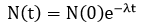Where N(0) is the initial number of unstable nuclei of a certain species, and λ is the disintegration constant which depends on the particular unstable species.

The rate of disintegration at any time ‘t’ is called activity: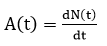The activity of a radioactive material at each moment is proportional to the number of unstable nuclei at that moment: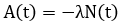By combining the above formulae, the law of exponential decay can be obtained: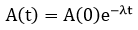Where A(0) is the activity at the moment zero (initial activity).

The unit of measure for activity is disintegration per second. To honour the discoverer of radioactivity (Henri Becquerel, 1896), the unit of activity (disintegration per second) was named the becquerel and the notation used is Bq.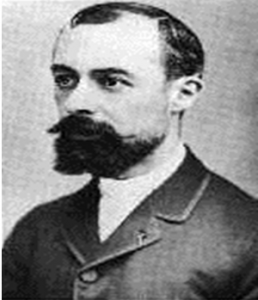###### Henri Becquerel (1852-1908)

Historically, another unit was used -the curie- noted Ci. It was named after Marie and Pierre Curie, the discoverers of Radium and Polonium. One curie (or 1 Ci) is the activity of 1 gram of pure Radium. This activity is equal to 3.7 x 1010 disintegration per second, or 3.7 x 1010 Bq.###### Marie Curie (1867-1934)            Pierre Curie (1859-1906)

The curie is a rather large activity for typical usage at U of T, and the becquerel is rather small. Therefore, Bq multiples and Ci sub-multiples are used. An example of the transformation between the two is given below:

### 2.9 Half-life

An important characteristic of each unstable nuclear species is the time it takes for the number of unstable nuclei to decrease to one half. This is called the “half-life” of the radionuclide. The half-life (T1/2) and the disintegration constant (λ) are connected by the following relationship: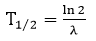where ln is the natural logarithm
With the half-life, the equation for radioactive decay (decrease in activity):Usually, radionuclide tables give the value for T1/2.

#### Example 2.9a:

The half-life of P-32 is 14.3 days. If 250 mCi (9.25 MBq) are bought and used after approximately 6 weeks (more precisely, after 43 days) the activity will be only: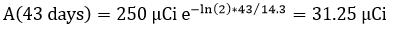* This is 8 times less than was initially bought. Therefore, it is better to order the radionuclide as close as possible to the date of use.

#### Example 2.9b:

The half-life of C-14 is 5730 years. Therefore, in the same interval as in example 2.9A (6 weeks), the activity of 1 mCi (37 MBq) of C-14 will practically not change at all: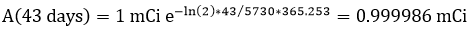*Go to the Periodic Table and click on the links to a few elements to get information on the most common radioisotopes, their half-lifes, decay modes, and the energy (in MeV) of the most important emitted radiation.